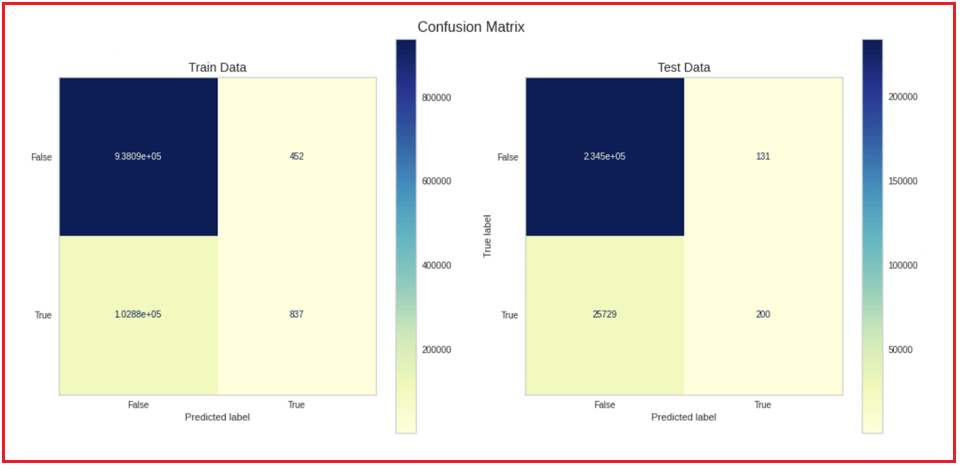# SVMs in Machine Learning

## Linear and Non-Linear SVMs in Machine Learning

In this article, I am going to discuss Linear and Non-Linear SVMs in Machine Learning with Examples. Please read our previous article where we discussed the Naive Bayes Algorithm in Machine Learning with Examples.

##### Introduction to SVMs

We’ll learn practically everything there is to know about Support Vector Machine, or simply SVM, a supervised machine learning technique that can be used for both classification and regression.

SVMs (Support Vector Machines) are one of the most often used and discussed machine learning techniques. The goal of SVM is to find a hyperplane in an N-dimensional space (N-Number of features) that categorizes data points clearly.

The Support Vector Machine is a variant of the maximum margin classifier. This classifier is straightforward; however, it can’t be used on most datasets because the classes must be divided by a linear boundary. However, it explains how the SVM works. Now let’s understand the two main terms of SVM – hyperplane and support vectors.

##### What is a Hyperplane?

A hyperplane is a flat affine subspace of dimension N-1 in an N-dimensional space. In 2D space, a hyperplane will appear as a line, whereas in 3D space, it will seem as a flat plane. In its most basic form, a hyperplane is a decision boundary that aids in the classification of data points.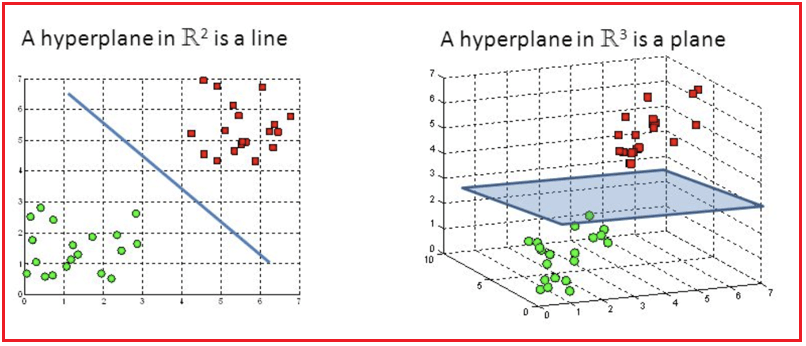There are numerous hyperplanes from which to choose in order to split two classes of data points. Our goal is to discover a plane with the greatest margin, or the greatest distance between data points from both classes, as shown in the diagram below.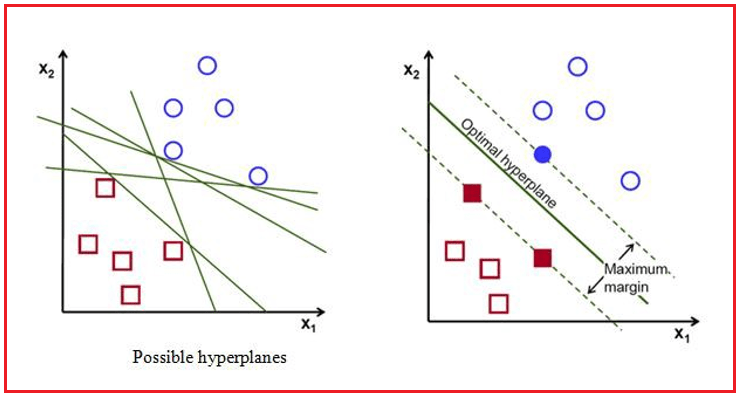Note – The number of features in a dataset determines the dimension of the hyperplane.

##### What are Support Vectors in Machine Learning?

The data points nearest to or on the hyperplane are called Support Vectors, and they influence the hyperplane’s position and orientation. We maximize the classifier’s margin by using these support vectors, and eliminating these support vectors will affect the hyperplane’s position. These are the points that assist us in the development of SVM.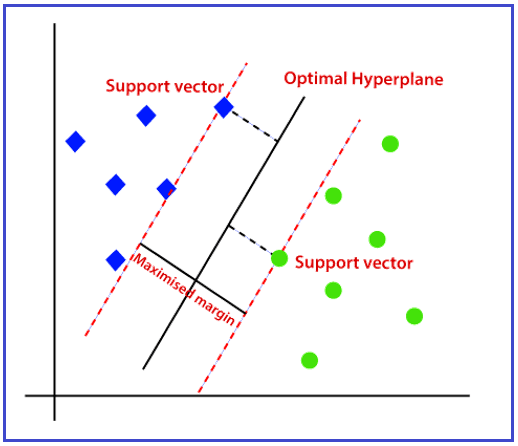The hyperplane is equidistant from the Support Vectors. They’ve termed support vectors because as their location changes, so does the hyperplane. This indicates that the hyperplane is solely determined by the support vectors and is unaffected by any other observations.

##### Linear SVMs in Machine Learning

If a separating boundary/hyperplane can readily be formed to distinguish the multiple class groups, the data points are considered to be linearly separable. Linear machine learning classifiers, such as logistic regression, are commonly used with linearly separable data points.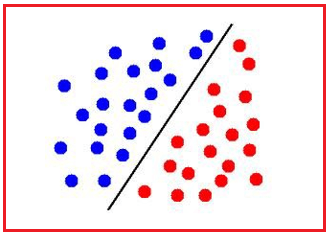We can express a hyperplane mathematically as –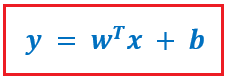where the output y denotes whether it belongs to the positive or negative class. w is the coefficient of x, where x represents the input data, as well as the matrix defining the plane’s parameters. The hyperplane’s intercept is represented by b.

When we have a large number of multidimensional features, such as p, the equation becomes: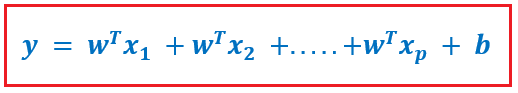We expect the classifier equation’s output to be either a positive number indicating that the data point belongs to a positive class or a negative number indicating that the data point belongs to a negative class in this example. The output of the classifier would be zero if any point exactly on the decision boundary, and hence the decision boundary equation is: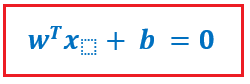Hence, the equations for hyperplane can be expressed as –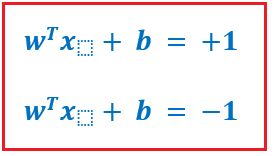Graphically these equations will look something like this –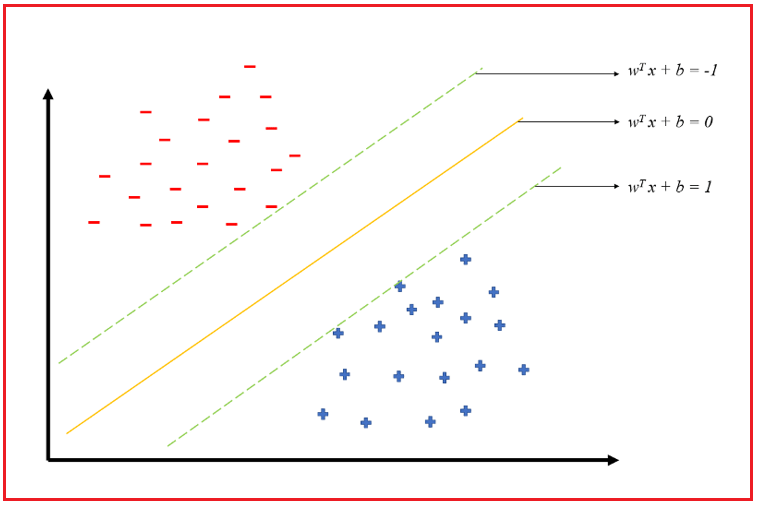##### Non-Linear SVMs in Machine Learning
###### What if the data isn’t split in a linear fashion?

Take a look at the graphic below, where the data is non-linearly split; obviously, we can’t identify the data points using a straight line. So, what are our options for resolving this issue?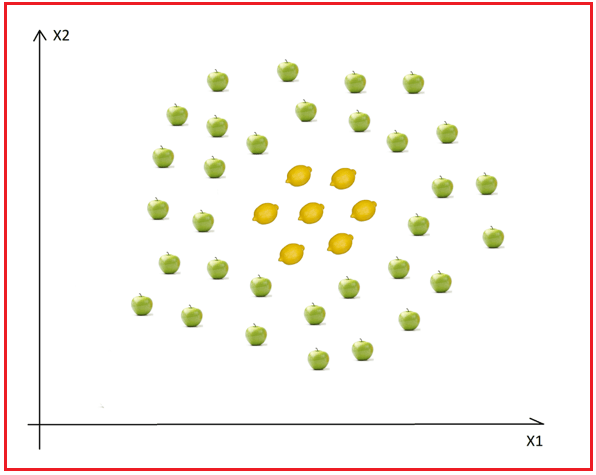##### The Kernel Trick will be used!

When data collection is inseparable from the present dimensions, the primary idea is to add another dimension to see if the data can be separated. Consider this: the example above is in 2D, and the apples and lemons are inseparable; but, in 3D, there may be a gap between the apples and the lemons, or a level differential, such that the lemons are on level one and the apples are on level two. In this scenario, a separating hyperplane (a plane in 3D) between levels 1 and 2 is simple to draw.

SVM deftly re-represents non-linear data points using any of the kernel functions in such a way that the data appears to have been transformed, then determines the best separating hyperplane. The data points, on the other hand, have not been modified; they have remained unchanged. This is why it’s known as a “kernel trick.” This is how 1-D and 2-D data can be transformed into higher-dimensional data.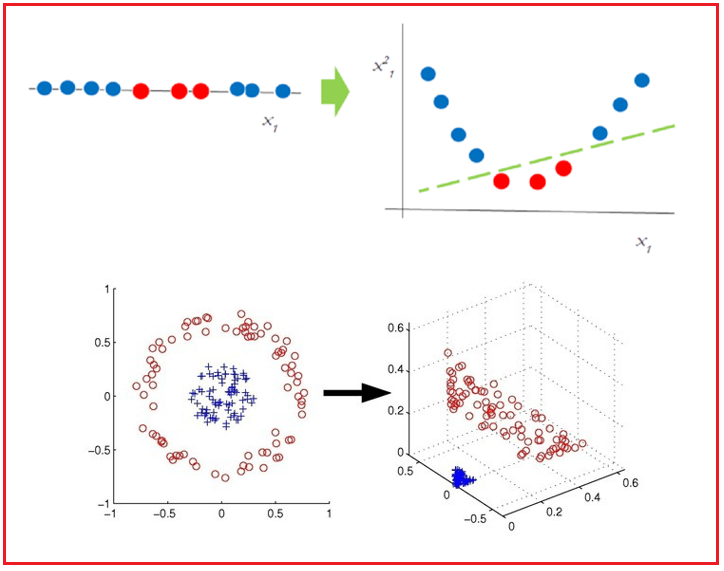The kernel technique allows you to use kernel functions to calculate relationships between data points and portray the data more efficiently with less processing. Kernelized models are models that use this technique.

##### Kernel SVMs in Machine Learning

When creating a hyperplane for linearly separable data points, SVM is simple. When the data is non-linearly separable, however, it becomes much more difficult. SVM kernels aid in the conversion of low-dimensional non-linearly separable data points into high-dimensional linearly separable data points. SVM kernels are divided into three categories:

##### Polynomial Function-

This uses the dot product to turn data points into an ‘n-dimension,’ where n can be any value between 2 and 3, i.e. the transformation will be either a squared product or higher. As a result, the new converted points are used to represent data in higher-dimensional space.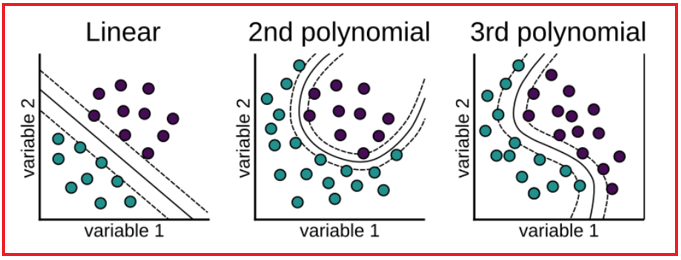##### Sigmoid Function-

It’s also known as the hyperbolic tangent function (Tanh), and it’s used as an activation function in neural networks. This function is used to classify images.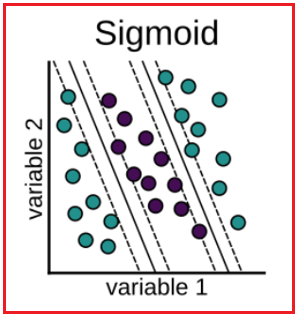This function works in the same way as a ‘weighted closest neighbor model.’ It changes data by describing it in infinite dimensions and then classifying it using the weighted nearest neighbor (observation with the greatest influence on the new data point). Either a Gaussian or a Laplace radial function can be used. This is determined by the gamma hyperparameter. This is the kernel that is most usually used.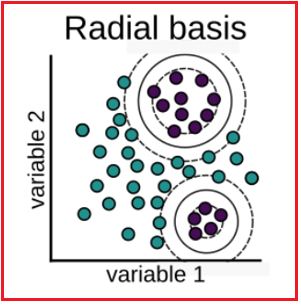### Case Study on Retail

##### Problem Statement

Shopping is a Canadian retail store that sells a variety of things to its clients. They have household-level transactions from 2,500 frequent shopper homes over a two-year period.

They want to figure out who is churning, why they’re churning, and who is likely to churn in the future. For business growth, they wish to increase their customer service and marketing approach. They employed a group of Data Scientists to help them with this challenge. Take into account that it’s you.

###### Importing Libraries
```# For Panel Data Analysis
import pandas as pd

# For Numerical Python
import numpy as np

# For Random seed values
from random import randint

# For Scientifc Python
from scipy import stats

# For Data Visualization
import matplotlib.pyplot as plt
%matplotlib inline
import seaborn as sns

# For Encoding Categorical Features
from category_encoders import TargetEncoder
from sklearn.preprocessing import OneHotEncoder, LabelEncoder

# For Feature Selection
from sklearn.feature_selection import SelectFromModel

# For Feature Importances
from yellowbrick.model_selection import FeatureImportances

# For metrics evaluation
from sklearn.metrics import precision_recall_curve, classification_report, plot_confusion_matrix

# To handle class imbalance problem
from imblearn.over_sampling import SMOTE

# For Data Modeling
from sklearn.model_selection import train_test_split, GridSearchCV
from sklearn.linear_model import LogisticRegression
from sklearn.ensemble import RandomForestClassifier
from sklearn.linear_model import LinearSVC

# To Disable Warnings
import warnings
warnings.filterwarnings(action = "ignore")

print('Shape:', data.shape)
```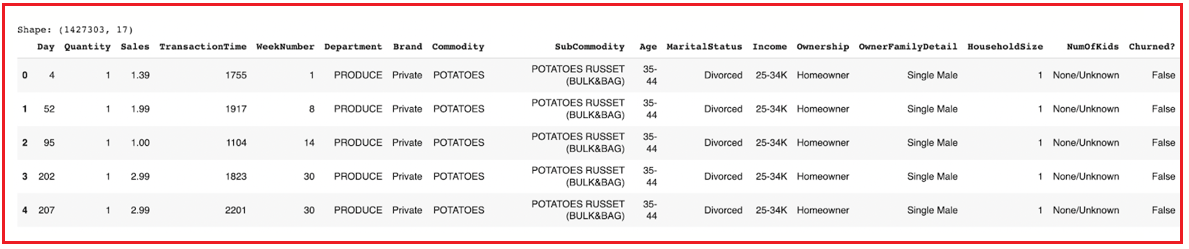##### Data Description
```print('Described Column Length:', len(data.describe().columns))
data.describe()
```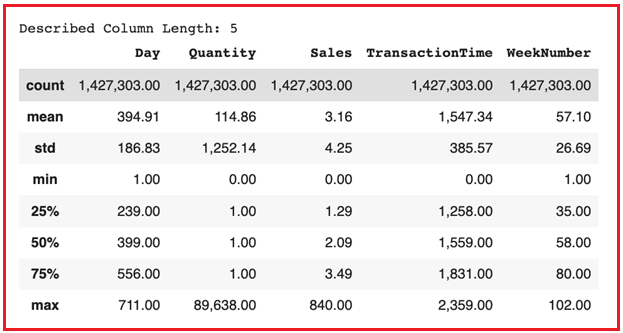`data.info(verbose = True, memory_usage = 'deep', null_counts = True)`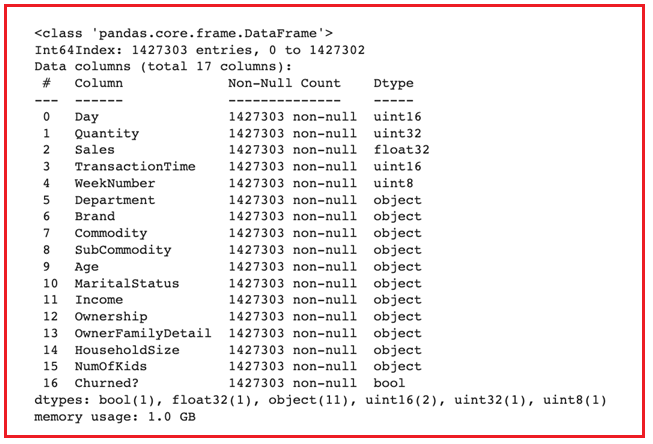```num_feature = []

for i in data.columns.values:
if ((data[i].dtype == np.uint8) | (data[i].dtype == np.uint16) | (data[i].dtype == np.uint32) | (data[i].dtype == np.float32) | (data[i].dtype == bool)):
num_feature.append(i)

print('Total Numerical Features:', len(num_feature))
print('Features:', num_feature)
```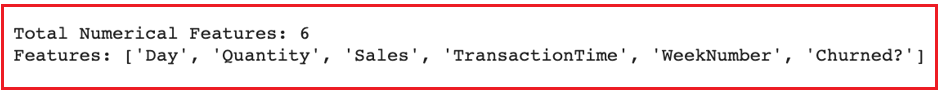```before_shape = data.shape
print('Data Shape [Before]:', before_shape)

data.drop_duplicates(inplace = True)

after_shape = data.shape
print('Data Shape [After]:', after_shape)

drop_nums = before_shape - after_shape
drop_percent = np.round(drop_nums / before_shape, decimals = 2) * 100

print('Drop Ratio:', drop_percent, '%')
```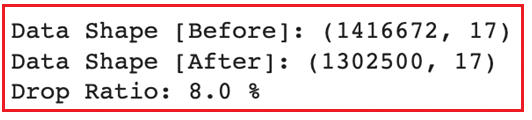##### Q. What is the proportion of churned customers vs non-churned customers?
```print('Customers who are Non-Churnable:', data['Churned?'].value_counts())
print('Customers who are Churnable:', data['Churned?'].value_counts())

space = np.ones(2)/10
data['Churned?'].value_counts().plot(kind = 'pie', explode = space, fontsize = 14, autopct = '%3.1f%%', wedgeprops = dict(width=0.15),
shadow = True, startangle = 160, figsize = [15, 8], legend = True)
plt.legend(['False', 'True'])
plt.ylabel('Churned?')
plt.title('Churned vs Non-Churned Customers', size = 14)
plt.show()
```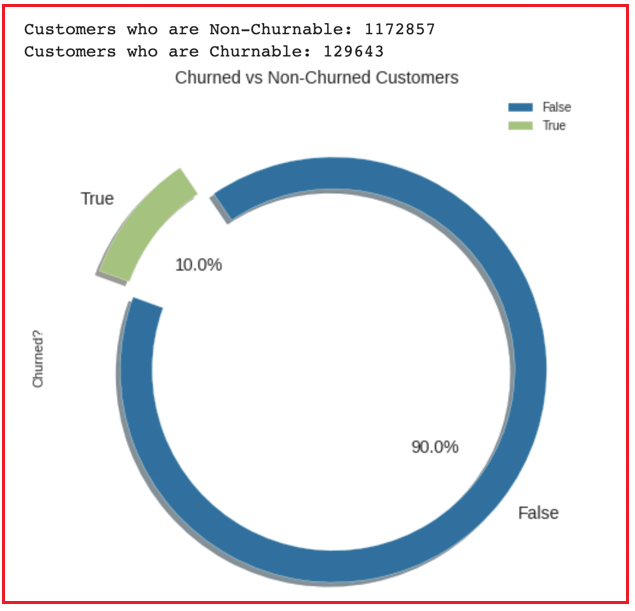##### Q. What is the frequency distribution of the Department?
```first_20 = data['Department'].value_counts()[0:20]
last_20 = data['Department'].value_counts()[20:40]

figure = plt.figure(figsize = [15, 8])
plt.subplot(1, 2, 1)
sns.barplot(x = first_20.values, y = first_20.index)
plt.xlabel('Frequency', size = 14)
plt.ylabel('Department', size = 14)
plt.subplot(1, 2, 2)
sns.barplot(x = last_20.values, y = last_20.index)
plt.xlabel('Frequency', size = 14)
plt.suptitle(t = 'Frequency Distribution of Department', size = 16,  y = 1.05)
plt.show()
```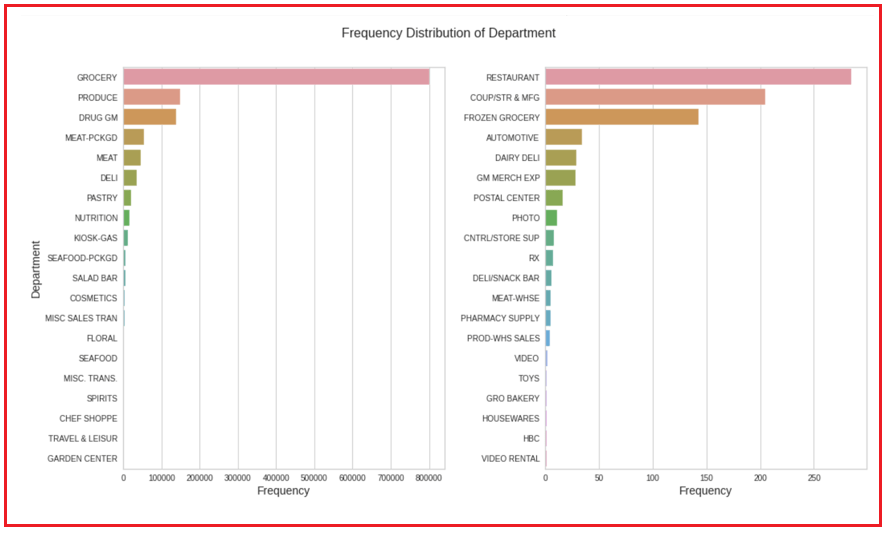```# Dummy Encoding -> Brand, MaritalStatus, Ownership, OwnerFamilyDetail, HouseholdSize, NumOfKids
data = pd.get_dummies(data = data, columns = ['Brand', 'MaritalStatus', 'Ownership', 'OwnerFamilyDetail', 'HouseholdSize', 'NumOfKids'])

# Label Encoding -> Age
le = LabelEncoder()
data['Age'] = le.fit_transform(data['Age'])

# Performing Target Encoding -> Department, Commodity, SubCommodity, Income
te = TargetEncoder(cols = ['Department', 'Commodity', 'SubCommodity', 'Income'])
te_data = te.fit_transform(X = data[['Department', 'Commodity', 'SubCommodity', 'Income']], y = data['Churned?'])

# Mapping Target Encoding Features back to original data
data['Department'] = te_data['Department']
data['Commodity'] = te_data['Commodity']
data['SubCommodity'] = te_data['SubCommodity']
data['Income'] = te_data['Income']

X = data[selected_feat]
y = data['Churned?']
X_train, X_test, y_train, y_test = train_test_split(X, y, test_size = 0.2, random_state = 42, stratify = y)

print('Training Data Shape:', X_train.shape, y_train.shape)
print('Testing Data Shape:', X_test.shape, y_test.shape)
```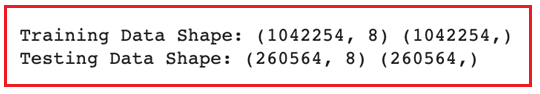```log  = LogisticRegression(random_state = 42, class_weight = 'balanced')
log.fit(X_train, y_train)

y_train_pred_count = log.predict(X_train)
y_test_pred_count = log.predict(X_test)

y_train_pred_proba = log.predict_proba(X_train)
y_test_pred_proba = log.predict_proba(X_test)

fig, (ax1, ax2) = plt.subplots(nrows = 1, ncols = 2, sharex = False, figsize=(15, 7))
plot_confusion_matrix(estimator = log, X = X_train, y_true = y_train, values_format = '.7g', cmap = 'YlGnBu', ax = ax1)
plot_confusion_matrix(estimator = log, X = X_test, y_true = y_test, values_format = '.7g', cmap = 'YlGnBu', ax = ax2)
ax1.set_title(label = 'Train Data', size = 14)
ax2.set_title(label = 'Test Data', size = 14)
ax1.grid(b = False)
ax2.grid(b = False)
plt.suptitle(t = 'Confusion Matrix', size = 16)
plt.show()
```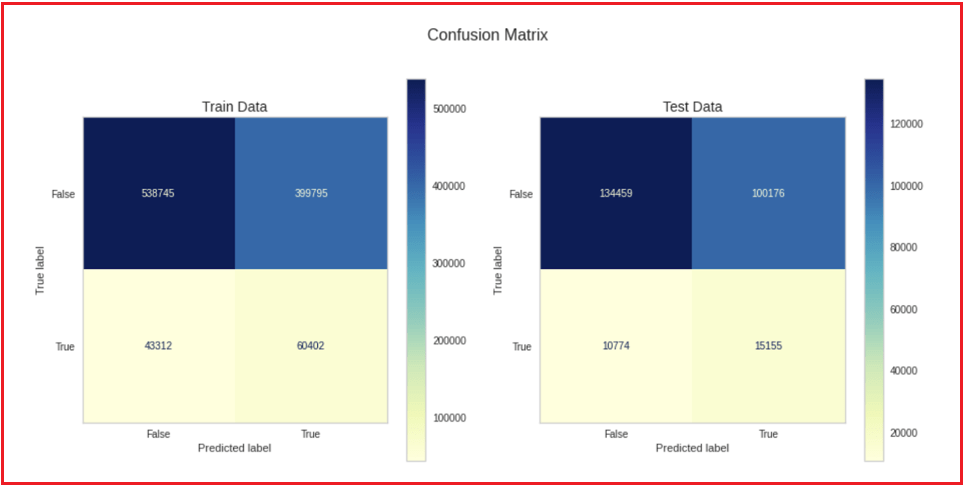```rfc = RandomForestClassifier(n_estimators = 100, n_jobs = -1, class_weight = 'balanced', random_state = 42)
rfc.fit(X_train, y_train)

y_train_pred_count = rfc.predict(X_train)
y_test_pred_count = rfc.predict(X_test)

y_train_pred_proba = rfc.predict_proba(X_train)
y_test_pred_proba = rfc.predict_proba(X_test)

fig, (ax1, ax2) = plt.subplots(nrows = 1, ncols = 2, sharex = False, figsize=(15, 7))
plot_confusion_matrix(estimator = rfc, X = X_train, y_true = y_train, values_format = '.7g', cmap = 'YlGnBu', ax = ax1)
plot_confusion_matrix(estimator = rfc, X = X_test, y_true = y_test, values_format = '.7g', cmap = 'YlGnBu', ax = ax2)
ax1.set_title(label = 'Train Data', size = 14)
ax2.set_title(label = 'Test Data', size = 14)
ax1.grid(b = False)
ax2.grid(b = False)
plt.suptitle(t = 'Confusion Matrix', size = 16)
plt.show()
```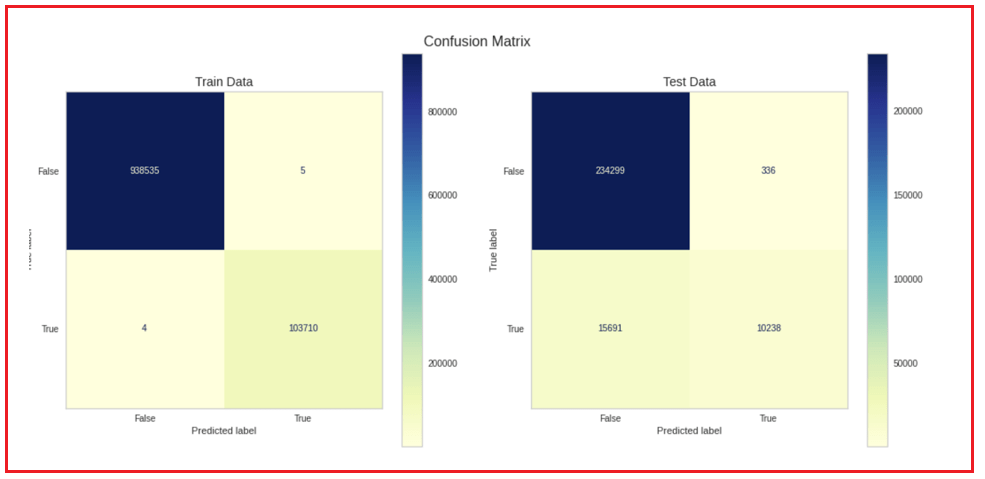```svc = LinearSVC(random_state = 42, n_jobs = -1)
svc.fit(X_train, y_train)

y_train_pred_count = svc.predict(X_train)
y_test_pred_count = svc.predict(X_test)

y_train_pred_proba = svc.predict_proba(X_train)
y_test_pred_proba = svc.predict_proba(X_test)

fig, (ax1, ax2) = plt.subplots(nrows = 1, ncols = 2, sharex = False, figsize=(15, 7))
plot_confusion_matrix(estimator = svc, X = X_train, y_true = y_train, values_format = '.7g', cmap = 'YlGnBu', ax = ax1)
plot_confusion_matrix(estimator = svc, X = X_test, y_true = y_test, values_format = '.7g', cmap = 'YlGnBu', ax = ax2)
ax1.set_title(label = 'Train Data', size = 14)
ax2.set_title(label = 'Test Data', size = 14)
ax1.grid(b = False)
ax2.grid(b = False)
plt.suptitle(t = 'Confusion Matrix', size = 16)